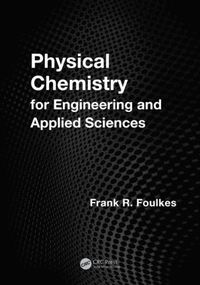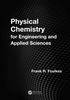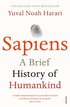Fler böcker inom
Format
Inbunden (Hardback)
Språk
Engelska
Antal sidor
704
Utgivningsdatum
2012-09-12
Förlag
CRC Press Inc
Medarbetare
Foulkes, F. R.
Illustratör/Fotograf
black and white 159 Illustrations 89 Tables black and white
Illustrationer
89 Tables, black and white; 159 Illustrations, black and white
Dimensioner
254 x 184 x 38 mm
Vikt
1338 g
Antal komponenter
1
Komponenter
68:B&W 7 x 10 in or 254 x 178 mm Case Laminate on White w/Gloss Lam
ISBN
9781466518469

Du kanske gillarPhysical Chemistry for Engineering and Applied Sciences

Inbunden Engelska, 2012-09-12
1179
Skickas inom 5-8 vardagar.
Fri frakt inom Sverige för privatpersoner.
Finns även som
• Laddas ned direkt
1089
Physical Chemistry for Engineering and Applied Sciences is the product of over 30 years of teaching first-year Physical Chemistry as part of the Faculty of Applied Science and Engineering at the University of Toronto. Designed to be as rigorous as compatible with a first-year student's ability to understand, the text presents detailed step-by-step derivations of the equations that permit the student to follow the underlying logic and, of equal importance, to appreciate any simplifying assumptions made or mathematical tricks employed. In addition to the 600 exercises and end-of-chapter problems, the text is rich in worked non-trivial examples, many of which are designed to be inspiring and thought-provoking. Step-by-step derivation of all equations enables the student to smoothly follow the derivation by sight, and can be understood relatively easily by students with moderate skills and backgrounds in mathematics. Clear and accessible, Physical Chemistry for Engineering and Applied Sciences includes: The answers to all of the 112 worked examples, 99 exercises following many of the worked examples, and 496 end-of-chapter problems Topics not normally seen in introductory physical chemistry textbooks (ionic reaction rates, activities and activity coefficients) or not regularly explained in much detail (electrochemistry, chemical kinetics), with an eye on industrial applications Special appendices that provide detailed explanations of basic integration and natural logarithms for students lacking a background in integral calculus An in-depth chapter on electrochemistry, in which activities and activity coefficients are used extensively, as required for accurate calculations

Passar bra ihop

1.2. +
3.De som köpt den här boken har ofta också köpt Sapiens av Yuval Noah Harari (häftad).

Kundrecensioner

Har du läst boken? Sätt ditt betyg »

Fler böcker av Frank R Foulkes

• Thermodynamics Step-By-Step

Frank R Foulkes

Most thermodynamics textbooks are lacking in the more detailed chemical thermodynamics that are important to engineering and applied sciences and often include too much material, emphasizing breadth rather than depth. A better approach is to empha...

Innehållsförteckning

THINGS YOU SHOULD KNOW BUT PROBABLY FORGOT Basic Definitions SI Units Dalton's Atomic Theory Stoichiometry Equivalent Weight Amount of Substance: the Mole Avogadro's Hypothesis Conservation of Mass Conservation of Charge Atomic Mass Scales STATES OF MATTER AND THE PROPERTIES OF GASES The Three States of Matter Pressure Archimedes' Principle Temperature THE IDEAL GAS The Ideal Gas Equation of State Molar Volumes Combined Gas Equation Dalton's Law of Partial Pressures Mole Fractions Partial Volumes THE KINETIC THEORY OF GASES Postulates Simplified Derivation of the Ideal Gas Law The Meaning of Pressure The Meaning of Temperature Diffusion and Effusion The Speeds of Gas Molecules Effect of Pressure on Speed Distribution of Molecular Speeds The Maxwell-Boltzmann Distribution as an Energy Distribution Fraction of Molecules Having E > E' Concluding Remarks REAL GASES Real Gases Isotherms for Real Gases Equations of State for Real Gases The Virial Equation The Van der Waals Equation Liquefaction of Gases THERMODYNAMICS (I) Thermodynamics Definitions Used In Thermodynamics Work PV-Work Maximum Work Obtainable from the Isothermal Expansion of an Ideal Gas Reversible Processes THERMODYNAMICS (II) Internal Energy (U) and the First Law of Thermodynamics State Functions Work and Heat Are Not State Functions Q and W Have Algebraic Signs Another Look At PV-Work Chemical Reaction in a Constant Volume System The Heat Capacity of a Single Phase System U for the Isothermal Expansion of an Ideal Gas The Internal Energy of a Monatomic Ideal Gas THERMODYNAMICS (III) Enthalpy (H) Constant Pressure Processes Thermochemistry H for Fusion (Melting) and Freezing H for Vaporization and Condensation H for Sublimation Ionization Enthalpies Electron Affinities Bond Enthalpies THERMODYNAMICS (IV) The Standard State for Chemical Reactions Hess's Law of Constant Heat Summation Standard Enthalpies of Formation Variation of H with Temperature at Constant Pressure THERMODYNAMICS (V) Spontaneous Processes Entropy (S) S for the Isothermal Expansion of an Ideal Gas S for a Constant Pressure Heating or Cooling Process S for a Constant Volume Heating or Cooling Process S for a Reversible Phase Change Whenever a Real Process Takes Place Suniv Increases Trouton's Rule THERMODYNAMICS (VI) Absolute Entropies and the Third Law of Thermodynamics Dealing With Phase Transitions Entropy Changes for Chemical Reactions THERMODYNAMICS (VII) Gibbs Free Energy (G) Gibbs Free Energy Changes, "Other" Work, and Spontaneity Evaluation of GT,P G Degrees for a Chemical Reaction Thermodynamic Stability CHEMICAL EQUILIBRIUM (I) Equilibrium Constants The Reaction Quotient (Q) and Relative Activities (ai) Formulation of Equilibrium Constants Molar Free Energies GR for Chemical Reactions CHEMICAL EQUILIBRIUM (II) Calculations Using Thermodynamic Equilibrium Constants Effect of Catalyst on K Effect of Temperature on K Effect of Pressure on K PHASE EQUILIBRIUM (I) Phase Change and Gibbs Free Energy Phase Diagram for a Single Pure Substance The Phase Rule PHASE EQUILIBRIUM (II) Phase Diagram for CO2 Phase Diagram for Water The Clapeyron Equation Phase Diagram for Carbon Phase Diagram for Helium The Clausius-Clapeyron Equation MIXTURES Expressions for Concentration Partial Molar Volumes The Chemical Potential ( ) The Chemical Potential of a Component in an Ideal Gas Mixture More About Activities Ideal Liquid Solutions of Volatile Solutes: Raoult's Law Ideal Liquid Solutions of Non-Volatile Solutes Ideal Liquid Solutions of Two Volatile Liquids Real (Non-Ideal) Solutions Chemical Potential and Solvent Activity Ideal Dilute Solutions of Volatile Solutes: Henry's Law The Solubility of Gases Distillation The Lever Rule Liquid-Liquid Phase Diagrams Liquid-Solid Phase Diagrams Compound Formation COLLIGATIVE PROPERTIES Colligative Properties Vapor Pres